xEncyclopedia
In fluid mechanics
Fluid mechanics
Fluid mechanics is the study of fluids and the forces on them. Fluid mechanics can be divided into fluid statics, the study of fluids at rest; fluid kinematics, the study of fluids in motion; and fluid dynamics, the study of the effect of forces on fluid motion...

, added mass or virtual mass is the inertia
Inertia
Inertia is the resistance of any physical object to a change in its state of motion or rest, or the tendency of an object to resist any change in its motion. It is proportional to an object's mass. The principle of inertia is one of the fundamental principles of classical physics which are used to...

added to a system because an accelerating or decelerating body must move some volume
Volume
Volume is the quantity of three-dimensional space enclosed by some closed boundary, for example, the space that a substance or shape occupies or contains....

of surrounding fluid
Fluid
In physics, a fluid is a substance that continually deforms under an applied shear stress. Fluids are a subset of the phases of matter and include liquids, gases, plasmas and, to some extent, plastic solids....

as it moves through it, since the object and fluid cannot occupy the same physical space simultaneously. For simplicity this can be modeled as some volume of fluid moving with the object, though in reality "all" the fluid will be accelerated, to various degrees.

The dimensionless added mass coefficient is the added mass divided by the displaced fluid mass – i.e. fluid density
Density
The mass density or density of a material is defined as its mass per unit volume. The symbol most often used for density is ρ . In some cases , density is also defined as its weight per unit volume; although, this quantity is more properly called specific weight...

times the volume of the body. In general, the added mass is a second-order tensor
Tensor
Tensors are geometric objects that describe linear relations between vectors, scalars, and other tensors. Elementary examples include the dot product, the cross product, and linear maps. Vectors and scalars themselves are also tensors. A tensor can be represented as a multi-dimensional array of...

, relating the fluid acceleration vector to the resulting force
Force
In physics, a force is any influence that causes an object to undergo a change in speed, a change in direction, or a change in shape. In other words, a force is that which can cause an object with mass to change its velocity , i.e., to accelerate, or which can cause a flexible object to deform...

vector on the body.

The concept of added mass can be thought of as a classical physics analogue of the quantum mechanical concept of quasiparticle
Quasiparticle
In physics, quasiparticles are emergent phenomena that occur when a microscopically complicated system such as a solid behaves as if it contained different weakly interacting particles in free space...

s. It is, however, not to be confused with relativistic mass increase.

## History

Friedrich Bessel
Friedrich Bessel
-References:* John Frederick William Herschel, A brief notice of the life, researches, and discoveries of Friedrich Wilhelm Bessel, London: Barclay, 1847 -External links:...

proposed the concept of added mass in 1828 to describe the motion of a pendulum in a fluid. The period of such a pendulum increased relative to its period in a vacuum (even after accounting for buoyancy effects), indicating that the surrounding fluid increased the effective mass of the system.

## Virtual mass force

Unsteady forces due to a change of the relative velocity of a body submerged in a fluid can be divided into two parts: the virtual mass effect and the Basset force
Basset force
In a body submerged in a fluid, unsteady forces due to acceleration of that body with respect to the fluid, can be divided into two parts: the virtual mass effect and the Basset force....

.

The origin of the force is that the fluid will gain kinetic energy at the expense of the work done by an accelerating submerged body.

It can be shown that the virtual mass force, for a spherical particle submerged in an inviscid, incompressible fluid is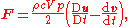where bold symbols denote vectors,is the fluid flow velocity
Flow velocity
In fluid dynamics the flow velocity, or velocity field, of a fluid is a vector field which is used to mathematically describe the motion of a fluid...

,is the spherical particle velocity,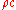is the mass density of the fluid
Fluid
In physics, a fluid is a substance that continually deforms under an applied shear stress. Fluids are a subset of the phases of matter and include liquids, gases, plasmas and, to some extent, plastic solids....

(continuous phase),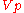is the volume of the particle, and D/Dt denotes the material derivative.

The origin of the notion "virtual mass" becomes evident when we take a look at the momentum equation for the particle.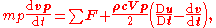where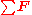is the sum of all other force terms on the particle, such as gravity, pressure gradient
In atmospheric sciences , the pressure gradient is a physical quantity that describes in which direction and at what rate the pressure changes the most rapidly around a particular location. The pressure gradient is a dimensional quantity expressed in units of pressure per unit length...

, drag
Drag (physics)
In fluid dynamics, drag refers to forces which act on a solid object in the direction of the relative fluid flow velocity...

, lift
Lift (force)
A fluid flowing past the surface of a body exerts a surface force on it. Lift is the component of this force that is perpendicular to the oncoming flow direction. It contrasts with the drag force, which is the component of the surface force parallel to the flow direction...

, Basset force
Basset force
In a body submerged in a fluid, unsteady forces due to acceleration of that body with respect to the fluid, can be divided into two parts: the virtual mass effect and the Basset force....

, etc.

Moving the derivative of the particle velocity from the right hand side of the equation to the left we get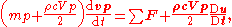so the particle is accelerated as if it had an added mass of half the fluid it displaces, and there is also an additional force contribution on the right hand side due to acceleration of the fluid.

## Applications

The added mass can be incorporated into most physics equations by considering an effective mass as the sum of the mass and added mass. This sum is commonly known as the "virtual mass".

A simple formulation of the added mass for a spherical body permits Newton's classical second law to be written in the form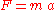becomes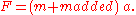One can show that the added mass for a sphere (of radius) is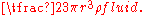For a general body, the added mass becomes a tensor
Tensor
Tensors are geometric objects that describe linear relations between vectors, scalars, and other tensors. Elementary examples include the dot product, the cross product, and linear maps. Vectors and scalars themselves are also tensors. A tensor can be represented as a multi-dimensional array of...

(referred to as the induced mass tensor), with components depending on the direction of motion of the body. Not all elements in the added mass tensor will have dimension mass, some will be mass × length and some will be mass × length2.

All bodies accelerating in a fluid will be affected by added mass, but since the added mass is dependent on the density of the fluid, the effect is often neglected for dense bodies falling in much less dense fluids. For situations where the density of the fluid is comparable to or greater than the density of the body, the added mass can often be greater than the mass of the body and neglecting it can introduce significant errors into a calculation.

For example, a spherical air bubble rising in water has a mass of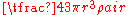but an added mass of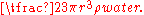Since water is approximately 800 times denser than air (at RTP
Standard conditions for temperature and pressure
Standard condition for temperature and pressure are standard sets of conditions for experimental measurements established to allow comparisons to be made between different sets of data...

), the added mass in this case is approximately 400 times the mass of the bubble.

### Naval architecture

These principles also apply to ships, submarines, and offshore platforms. In ship design, the energy required to accelerate the added mass must be taken into account when performing a sea keeping analysis. For ships, the added mass can easily reach ¼ or ⅓ of the mass of the ship and therefore represents a significant inertia
Inertia
Inertia is the resistance of any physical object to a change in its state of motion or rest, or the tendency of an object to resist any change in its motion. It is proportional to an object's mass. The principle of inertia is one of the fundamental principles of classical physics which are used to...

, in addition to frictional and wavemaking drag forces. Since added mass is a virtual mass and not a real mass, it is not taken into account for structural designs.

In aircraft, the added mass is not usually taken into account because the density of the air is so small.

• Basset force
Basset force
In a body submerged in a fluid, unsteady forces due to acceleration of that body with respect to the fluid, can be divided into two parts: the virtual mass effect and the Basset force....

• Response Amplitude Operator
Response amplitude operator
In the field of ship design and design of other floating structures, a response amplitude operator is an engineering statistic, or set of such statistics, that are used to determine the likely behaviour of a ship when operating at sea...

for the use of added mass in ship design
• Keulegan–Carpenter number
Keulegan–Carpenter number
In fluid dynamics, the Keulegan–Carpenter number, also called the period number, is a dimensionless quantity describing the relative importance of the drag forces over inertia forces for bluff objects in an oscillatory fluid flow. Or similarly, for objects that oscillate in a fluid at rest...

for a dimensionless parameter giving the relative importance of the drag
Drag (physics)
In fluid dynamics, drag refers to forces which act on a solid object in the direction of the relative fluid flow velocity...